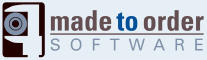# libsswf: sswf::Edges Class Reference

List of coordinates to draw shapes. More...

`#include <libsswf.h>`

Inheritance diagram for sswf::Edges:## Public Types

enum  { EDGE_BLOCK = 64 }

## Public Member Functions

Edges (void)
Initialize the Edges object.
void Save (Data &data, long &x, long &y)
Save the Edges in the Data buffer.
void Set (int index, const edge_t &edge)
Set an edge at the specified index.
void Set (const edge_t &edge)
Append the specified edge.
void Set (int index, long x, long y, long ctrl_x, long ctrl_y)
Append point (x, y) with control point (ctrl_x, ctrl_y) at position index.
void Set (long x, long y, long ctrl_x, long ctrl_y)
Append point (x, y) with control point (ctrl_x, ctrl_y).
void Set (long x, long y)
Append point (x, y).
virtual ~Edges ()
Clean up a set of edges.

## Private Member Functions

Edges (const Edges &edges)
Edgesoperator= (const Edges &edges)
void SaveEdge (Data &data, const edge_t &edge, long &x, long &y)

## Private Attributes

array_edge_t f_array
Vectors f_edges
int f_pos

## Classes

struct  array_edge_t
One set of edges; when full, allocate another. More...
class  edge_t
The basic structure used to hold the coordinates of an edge. More...

## Detailed Description

The Edges class represents an array of edges used to draw shapes.

An edge is one point on a curve and eventually one control point. All edges are specified in a two dimensional plane (i.e. x and y coordinates).

You can use the sswf::Edges::edge_t structure to setup a point and then add it to the Edges class (C++ only) or use a specialized function which will automatically create such a structure for you.

There is no limit to the number of points in an Edges class. However, every EDGE_BLOCK points, a new array is allocated to save more points.

Note that there are no tests on the edges. You need to ensure that they are valid for a Flash movie. It is strongly suggested that you do not use too large or too small a value. The edges values are in TWIPS (i.e. 1/20th of a pixel).

The control points are set to LONG_MIN so as to be ignored. Such a large value cannot be represented in Flash and it would be way too large anyway.

Warning:
Note that all the coordinates are specified as in Flash: the next coordinate is ADDED to the previous coordinate. Thus if you have three points:
```        (10, 32)
(40, 14)
(3, 100)
```

You want to pass to the edges the following coordinates:

```        (10, 32)
(40 - 10, 14 - 32)
(3 - (40 - 10), 100 - (14 - 32))
```

sswf::Edges::edge_t

## Member Enumeration Documentation

 anonymous enum

Enumerator:
 EDGE_BLOCK

## Constructor & Destructor Documentation

 Edges::Edges ( void )

The constructor ensures that the list of points is empty.

References f_pos.

 Edges::~Edges ( ) ` [virtual]`

This function ensures that the edges are being deleted from memory.

 sswf::Edges::Edges ( const Edges & edges ) ` [inline, private]`

## Member Function Documentation

 Edges& sswf::Edges::operator= ( const Edges & edges ) ` [inline, private]`

 void Edges::Save ( Data & data, long & x, long & y )

This function saves the array of edges in the specified Data buffer.

The x and y parameters are in/out coordinates used to know where these edges end. If you enter the function with x and y set to 0, then you will know exactly where the edges end as if the shape was starting at the origin. This is used to move to the next set of edges and close shapes.

Note:
The x and y coordinates have NO effect on what is being saved by this function.
Parameters:
 data The data buffer where the data is to be saved x The starting (in) and ending (out) horizontal coordinate y The starting (in) and ending (out) vertical coordinate

Referenced by sswf::TagShape::SaveShape().

 void Edges::SaveEdge ( Data & data, const edge_t & edge, long & x, long & y ) ` [private]`

 void Edges::Set ( int index, const edge_t & edge )

Copy the specified edge at the specified index.

If the index is set to -1, then the edge is appended to the existing list.

Parameters:
 index The index where the edge is to be saved or -1 to append edge A valid edge structure

 sswf::Edges::Set ( const edge_t & edge ) ` [inline]`

This function adds the specified edge at the end of the existing edges.

Parameters:
 edge The edge to append

References Set().

 sswf::Edges::Set ( int index, long x, long y, long ctrl_x, long ctrl_y ) ` [inline]`

Set a curve edge at the specified 'index' with coordinates (x, y) and control point (ctrl_x, ctrl_y).

This point overwrites the existing point at coordinate 'index'.

If index is set to -1, then the new point is added at the end (appended).

Parameters:
 index The position in the array of edges where the edge is saved x The horizontal coordinate y The vertical coordinate ctrl_x The horizontal control point coordinate ctrl_y The vertical control point coordinate

References Set().

 sswf::Edges::Set ( long x, long y, long ctrl_x, long ctrl_y ) ` [inline]`

Add a curve edge with coordinates (x, y) and control point (ctrl_x, ctrl_y).

This point is appended at the end of the existing list.

Parameters:
 x The horizontal coordinate y The vertical coordinate ctrl_x The horizontal control point coordinate ctrl_y The vertical control point coordinate

References Set().

 sswf::Edges::Set ( long x, long y ) ` [inline]`

Add a straight line edge with coordinates (x, y)

This point is appended at the end of the existing list.

Parameters:
 x The horizontal coordinate y The vertical coordinate

Referenced by sswf::TagShape::AddEdge(), sswf::TagShape::Save(), and Set().

## Member Data Documentation

 array_edge_t sswf::Edges::f_array` [private]`

Referenced by Save(), and Set().

 Vectors sswf::Edges::f_edges` [private]`

Referenced by Save(), and Set().

 int sswf::Edges::f_pos` [private]`

Referenced by Edges(), Save(), and Set().

The documentation for this class was generated from the following files:

Generated on Wed Mar 18 15:13:58 2009 for libsswf by1.5.5